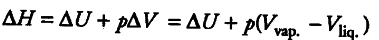# Determine the value of ∆H and ∆U for the reversible isothermal

Determine the value of ∆H and ∆U for the reversible isothermal evaporation of 90.0 g of water at 100° C. Assume that water vapour behave as an ideal gas and heat of evaporation of water is 540 cal.

∆H = 90.0 x 540 =48600 calVolume of liquid is negligible as compared to volume of vapour.
∆H = ∆U + { pV }_{ vap }
∆H = ∆U + nRT
= 48600 - 90/18 x 2 x 373(n = 90/18)
= 48600 - 3730 = 44870 cal

shouldnt dU be zero since it is an isothermal process?

Yes, I completely agree with u